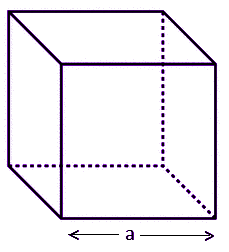# what is the area of a cube

All Questionswhat is the area of a cube

Surface area of cube is the sum of areas of all the faces of cube, that covers it. The formula for surface area is equal to six times of square of length of the sides of cube. It is represented by 6a2, where a is the side length of cube. It is basically the total surface area. Also, learn Volume Of A Cube.
When a cube is kept in a three-dimensional space, the area occupied by the sides of the cube in the space is called surface area of cube. In real world, we have been surrounded by many solid objects which have their own area as well volume. The area defines the region occupied by the objects and volume defines the space contained inside that object. The basic solid shapes or 3D shapes which we have learned till now in geometry are:

• Cube
• Cuboid
• Cylinder
• Cone
• Sphere

Surface Area of Cube Definition
The definition of surface area of a given cube states that if the total surface area is equal to the sum of all the areas of the faces of the cube. Since the cube has six faces, therefore, the total surface area of the cube will be equal to sum of all six faces of cube.
Since, the surface of the cube is in square shape. Hence, area of each face of the cube is equal to square of edge. Let the length of edge of cube is a.
Therefore, area of one face = a2    [By area of square formula]
There are total 6 faces. Therefore,
TSA of cube = a2 + a2 + a2 + a2 + a2 +a2
TSA of cube = 6a2
A cube consists of ‘n’ number of square units. Hence the space covered by these square units on the surface of the cube is the surface area. Basically, the surface area is the sum of all the area of all the shapes that cover the surface of the shape or object. In the case of a cube, there are 6 faces. So the surface area will be sum of all the area of six faces.
Let us derive the formula for surface area for a given cube, to solve problems based on it.
Surface Area of a Cube Formula
As per the definition of the cube, we know, the cube consists of 6 square faces. Let us consider, a cube whose length of the edges is ‘a’.Now, we know, by the formula of area of a square;
Area = Side2 = a2
Therefore, the total surface area of a cube = 6 × (area of each side)
= 6 × a2 = 6a2 Square Unit

TSA = 6 a2